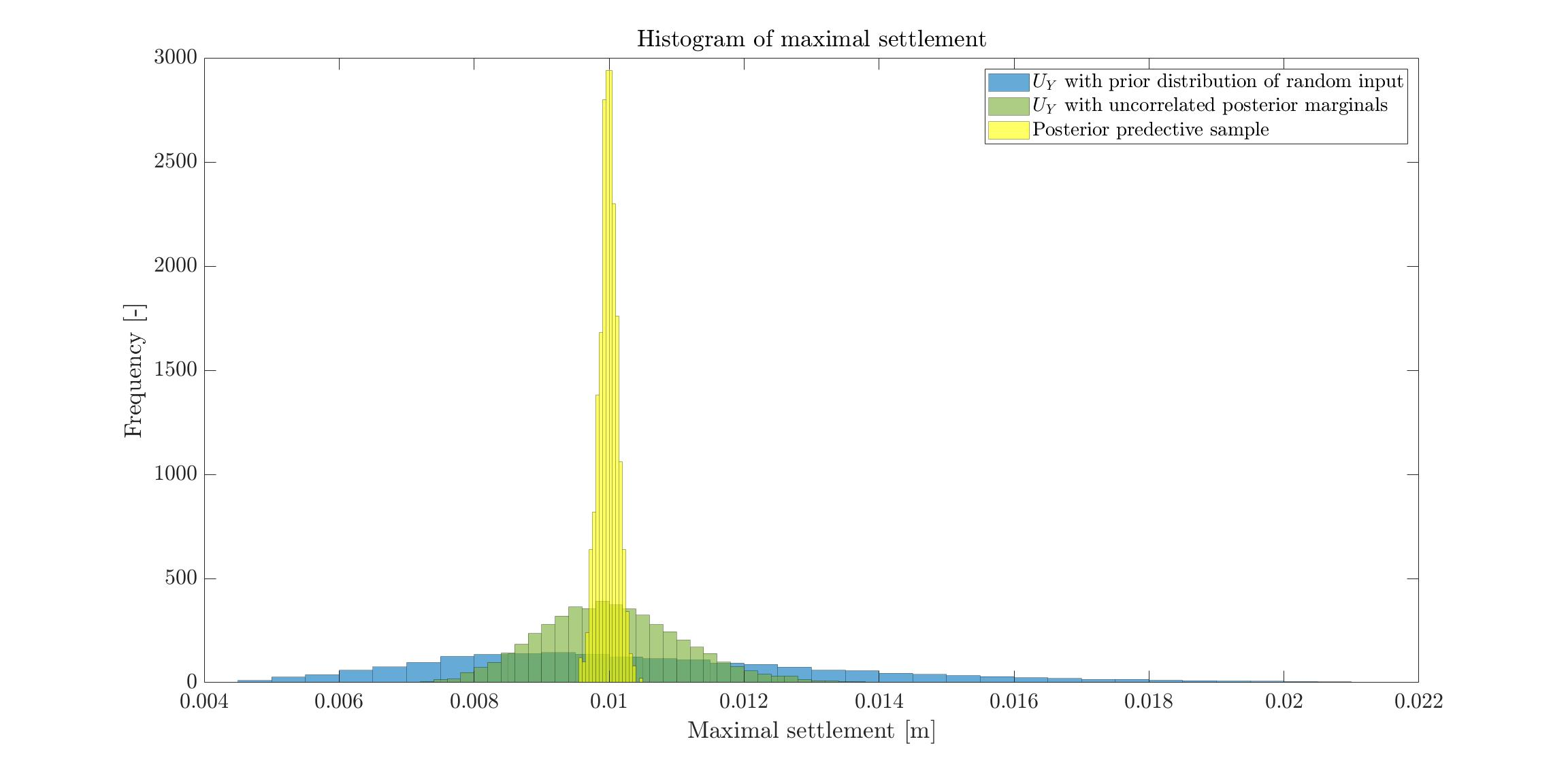# Forward reliability analysis using the posterior marginals given by the bayesian inversion

Hello,

I have the following situation. There is a foundation lying on a two layers soil. The Young’s moduli, E_1 and E_2, of the two soil layers are my random variables. The quantity of interest is the maximal settlement U_Y of the foundation under a given load F. Now say that in the deterministic case using mean values of E_1 and E_2 I calculate (with FE software) a settlement of 1 cm. But at the building site I observe a settlement of 1.5 cm. So I perform a bayesian inversion (I first build a PCE on the FE software, very small LOO error) in order to update my probabilistic input. As a result of the bayesian inversion I get the posterior marginals and the correlation matrix. Since the output U_Y is a function of all inputs that feed my FE software/PCE, U_Y \approx f(E_1,E_2), it is logic that my varibles are correlated in order to “match” the observation of 1.5 cm.

What I don’t understand is if for a forward reliability analysis I should use as probabilistic input the uncorrelated posterior marginals or the correlated posterior marginals as explained in Looking for documentation/example of using UQLab results of Bayesian inference for forward UQ or further Bayesian inference. The point is, in reality E_1 and E_2 have no correlation. There is no physical reason to have a correlation between them.

Dear Gil,

In my opinion, you should use the correlated posterior marginals since they should produce the more valid results.

• Even if initially the Young’s moduli E_1 and E_2 are really not correlated: Since you have the observation of a a settlement of 1.5 cm you should use for further computation samples (E_{1,i,}E_{2,i}) for (E_1,E_2) that produce approximation of this settlement, i.e. those pairs that “match” the observation of 1.5 cm. This is similar to the situation in Bayesian inversion : limit the correlation of posterior inputs.
And to get sample pairs of this kind, you need that the random variables representing E_1 and E_2 are correlated.
If you only use the uncorrelated posterior marginals, and use then the corresponding mean values to computed the settlement you may end up in s situation similar to the one in Example of Bayesian inference computation creating a model output at the mean of the parameter samples that is outside of the discrete posterior predictive support.

• Minor remark: I am not sure that one can really rule out thatt the Young’s moduli E_1 and E_2 are correlated for physical reasons: The two soil layers are connected at some interface such that one may have a material exchange between the layers through this interface changing the material properties of the soil near to the interface such that the effective Young’s moduli you seem to use in your computation may become correlated.

Greetings
Olaf

Dear Olaf,